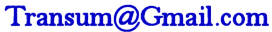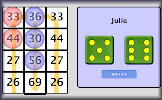# Differentiation

## Practise the technique of differentiating polynomials and other functions with this self marking exercise.

##### Level 1L 2L 3L 4L 5L 6L 7L 8L 9L 10L 11Exam-StyleDescriptionHelp

This is level 1: differentiate basic polynomials. You can earn a trophy if you get at least 9 questions correct and you do this activity online. Use the ^ key to type in a power or index and use the forward slash / to type a fraction. Press the right arrow key to end the power or fraction. Click the Help tab above for more.

 $$y=x^{4}$$ $$\frac{dy}{dx}=$$$$y=x^{3}$$ $$\frac{dy}{dx}=$$$$y=x^{2}$$ $$\frac{dy}{dx}=$$$$y=x$$ $$\frac{dy}{dx}=$$$$y=x^{3}+x^{2}$$ $$\frac{dy}{dx}=$$$$y=x^{4}-x^{3}$$ $$\frac{dy}{dx}=$$$$y=x+2$$ $$\frac{dy}{dx}=$$$$y=8x^{4}$$ $$\frac{dy}{dx}=$$$$y=8x$$ $$\frac{dy}{dx}=$$$$y=2$$ $$\frac{dy}{dx}=$$$$y=5x^{4}+8x^{3}-3$$ $$\frac{dy}{dx}=$$$$y=4x^{2}-5x+5$$ $$\frac{dy}{dx}=$$Check

This is Differentiation level 1. You can also try:
Level 2 Level 3 Level 4 Level 5 Level 6 Level 7 Level 8 Level 9 Level 10 Level 11

## Instructions

Try your best to answer the questions above. Type your answers into the boxes provided leaving no spaces. As you work through the exercise regularly click the "check" button. If you have any wrong answers, do your best to do corrections but if there is anything you don't understand, please ask your teacher for help.

When you have got all of the questions correct you may want to print out this page and paste it into your exercise book. If you keep your work in an ePortfolio you could take a screen shot of your answers and paste that into your Maths file.

## Transum.org

This web site contains over a thousand free mathematical activities for teachers and pupils. Click here to go to the main page which links to all of the resources available.

Please contact me if you have any suggestions or questions.## More Activities:

Mathematicians are not the people who find Maths easy; they are the people who enjoy how mystifying, puzzling and hard it is. Are you a mathematician?

Comment recorded on the 24 May 'Starter of the Day' page by Ruth Seward, Hagley Park Sports College:

"Find the starters wonderful; students enjoy them and often want to use the idea generated by the starter in other parts of the lesson. Keep up the good work"

Comment recorded on the 1 May 'Starter of the Day' page by Phil Anthony, Head of Maths, Stourport High School:

"What a brilliant website. We have just started to use the 'starter-of-the-day' in our yr9 lessons to try them out before we change from a high school to a secondary school in September. This is one of the best resources on-line we have found. The kids and staff love it. Well done an thank you very much for making my maths lessons more interesting and fun."

#### Remainder RaceA brilliant game involving chance and choice requiring an ability to calculate the remainder when a two digit number is divided by a single digit number. There are one and two player versions and the rules are inspired by the Royal Game of Ur.

There are answers to this exercise but they are available in this space to teachers, tutors and parents who have logged in to their Transum subscription on this computer.

A Transum subscription unlocks the answers to the online exercises, quizzes and puzzles. It also provides the teacher with access to quality external links on each of the Transum Topic pages and the facility to add to the collection themselves.

Subscribers can manage class lists, lesson plans and assessment data in the Class Admin application and have access to reports of the Transum Trophies earned by class members.

If you would like to enjoy ad-free access to the thousands of Transum resources, receive our monthly newsletter, unlock the printable worksheets and see our Maths Lesson Finishers then sign up for a subscription now:

Subscribe

## Go Maths

Learning and understanding Mathematics, at every level, requires learner engagement. Mathematics is not a spectator sport. Sometimes traditional teaching fails to actively involve students. One way to address the problem is through the use of interactive activities and this web site provides many of those. The Go Maths page is an alphabetical list of free activities designed for students in Secondary/High school.

## Maths Map

Are you looking for something specific? An exercise to supplement the topic you are studying at school at the moment perhaps. Navigate using our Maths Map to find exercises, puzzles and Maths lesson starters grouped by topic.

## Teachers

If you found this activity useful don't forget to record it in your scheme of work or learning management system. The short URL, ready to be copied and pasted, is as follows:

Do you have any comments? It is always useful to receive feedback and helps make this free resource even more useful for those learning Mathematics anywhere in the world. Click here to enter your comments.For All:

© Transum Mathematics :: This activity can be found online at:
www.Transum.org/go/?Num=55

## Description of Levels

CloseBefore beginning these exercises make sure you understand Indices really well.

Level 1 - Differentiate basic polynomials

Level 2 - Differentiate polynomials including negative and fractional indices

Level 3 - Find the gradient at the given point

Level 4 - Finding tangents and normals

Level 5 - Differentiate trigonometric functions

Level 6 - Differentiate exponential and natural logarithm functions

Level 7 - Differentiate using the chain rule

Level 8 - Differentiate using the product rule

Level 9 - Differentiate using the quotient rule

Level 10 - Interpreting derivatives and second derivatives, maxima, minima and points of inflection.

Level 11 - Differentiate simple functions parametrically

Exam Style questions are in the style of IB or A-level exam paper questions and worked solutions are available for Transum subscribers.

Integration - Exercises on indefinite and definite integration of basic algebraic and trigonometric functions.

Answers to this exercise are available lower down this page when you are logged in to your Transum account. If you don’t yet have a Transum subscription one can be very quickly set up if you are a teacher, tutor or parent.

## Example

The video above is from MathMateVideos.

## Mathematical Notation

Use the ^ key to type in a power or index then the right arrow or tab key to end the power.

For example: Type 3x^2 to get 3x2.

Use the forward slash / to type a fraction then the right arrow or tab key to end the fraction.

For example: Type 1/2 to get ½.

Fractions should be given in their lowest terms.

A square root sign (if required) should be typed in as \sqrt space then press the right arrow key after typing in the last term in the square root.

## Terminology and symbols

Please note that if $$y = f(x) = x^2$$ then the first differential can be shown in any of the following ways:

$$\frac{dy}{dx} = 2x$$ $$y' = 2x$$ $$f'(x) = 2x$$

## Differentiating Trigonometric Functions

$$\frac{d}{dx} (\sin x) = \cos x$$ $$\frac{d}{dx} (\cos x) = -\sin x$$ $$\frac{d}{dx} (\tan x) = \frac{1}{\cos^2 x}$$

## Differentiating Other Functions

$$\frac{d}{dx} (e^x) = e^x$$ $$\frac{d}{dx} ( \ln x) = \frac{1}{x}$$

In the following rules, $$u$$ and $$v$$ are functions of $$x$$.

## The Product Rule

$$\text{If} \quad y = uv \quad \text{then}$$ $$\frac{dy}{dx} = v\frac{du}{dx} + u\frac{dv}{dx}$$

## The Quotient Rule

$$\text{If} \quad y = \frac{u}{v} \quad \text{then}$$ $$\frac{dy}{dx} = \frac{v\frac{du}{dx} - u\frac{dv}{dx}}{v^2}$$

## The Chain Rule

$$\frac{dy}{dx} = \frac{dy}{du} \times \frac{du}{dx}$$